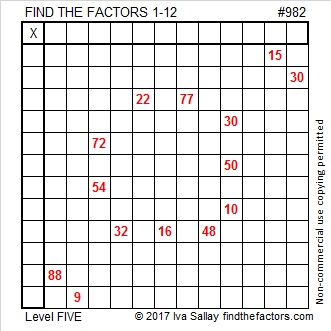# 1589 Candy Bars

### Today’s Puzzle:

Perhaps you can imagine that this level 4 puzzle looks like a couple of candy bars, one for you and one for me!### Factors of 1589:

Divisibility rules let us know quickly that 1589 is not divisible by 2, 3, or 5. Is it divisible by 7, the next prime number. We can apply the divisibility rule for 7 to find out:

1589 is divisible by 7 because
158 – 2(9) = 158 – 18 = 140, and 140 is divisible by 7.

• 1589 is a composite number.
• Prime factorization: 1589 = 7 × 227.
• 1589 has no exponents greater than 1 in its prime factorization, so √1589 cannot be simplified.
• The exponents in the prime factorization are 1 and 1. Adding one to each exponent and multiplying we get (1 + 1)(1 + 1) = 2 × 2 = 4. Therefore 1589 has exactly 4 factors.
• The factors of 1589 are outlined with their factor pair partners in the graphic below.### More about the Number 1589:

1589 is the difference of two squares two different ways:
795² – 794² = 1589, and
117² – 110² = 1589.

1589 is the sum of consecutive numbers in three different ways:
1589 is the sum of the two numbers from 794 to 795.
1589 is the sum of the seven numbers from 224 to 230.
1589 is also the sum of the fourteen numbers from 107 to 120,

# 1567 Peppermint Stick

### Today’s Puzzle:

Our mystery level puzzle looks like a sweet stick of Christmas candy. Will solving it be sweet or will it be sticky? You’ll have to try it yourself to know.### Factors of 1567:

• 1567 is a prime number.
• Prime factorization: 1567 is prime.
• 1567 has no exponents greater than 1 in its prime factorization, so √1567 cannot be simplified.
• The exponent in the prime factorization is 1. Adding one to that exponent we get (1 + 1) = 2. Therefore 1567 has exactly 2 factors.
• The factors of 1567 are outlined with their factor pair partners in the graphic below.How do we know that 1567 is a prime number? If 1567 were not a prime number, then it would be divisible by at least one prime number less than or equal to √1567. Since 1567 cannot be divided evenly by 2, 3, 5, 7, 11, 13, 17, 19, 23, 29, 31, or 37, we know that 1567 is a prime number.

### More about the Number 1567:

1567 is the sum of two consecutive numbers:
783 + 784 = 1567.

1567 is also the difference of two consecutive squares:
784² – 783² = 1567.

# 982 Red-Hot Cinnamon Candy

When I was a child I remember eating a red-hot cinnamon ball around the holidays. I really like cinnamon, but I wasn’t sure I liked how hot the candy was. I hope you enjoy today’s red-hot cinnamon candy puzzle.Print the puzzles or type the solution in this excel file: 12 factors 978-985

Now here’s something interesting about the number 982:

It is palindrome 292 in BASE 20 because 2(20²) + 9(20) + 2(1) = 982.

• 982 is a composite number.
• Prime factorization: 982 = 2 × 491
• The exponents in the prime factorization are 1 and 1. Adding one to each and multiplying we get (1 + 1)(1 + 1) = 2 × 2 = 4. Therefore 982 has exactly 4 factors.
• Factors of 982: 1, 2, 491, 982
• Factor pairs: 982 = 1 × 982 or 2 × 491
• 982 has no square factors that allow its square root to be simplified. √982 ≈ 31.336879